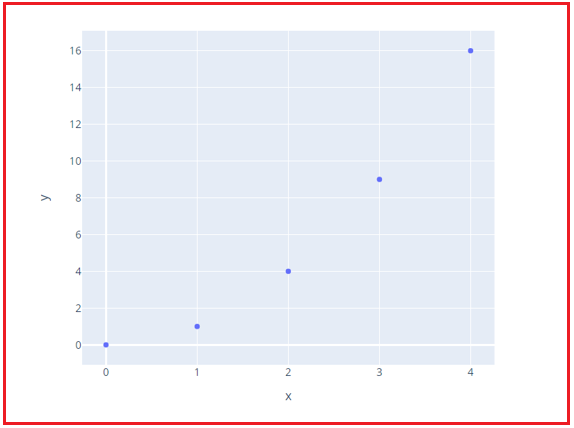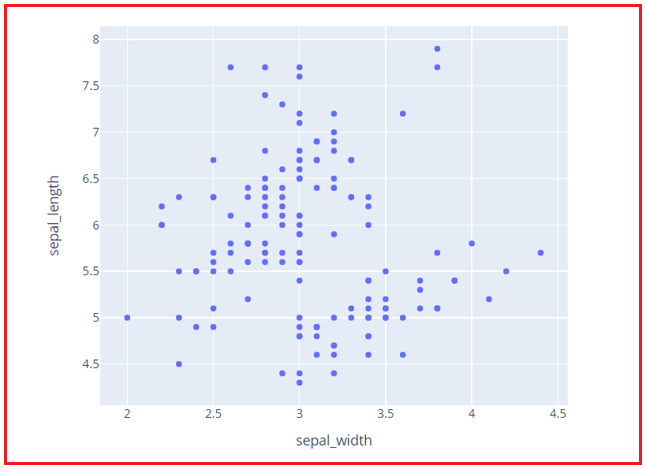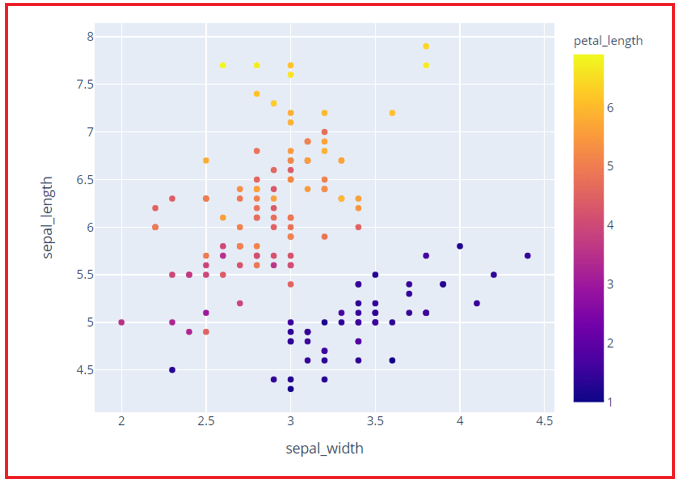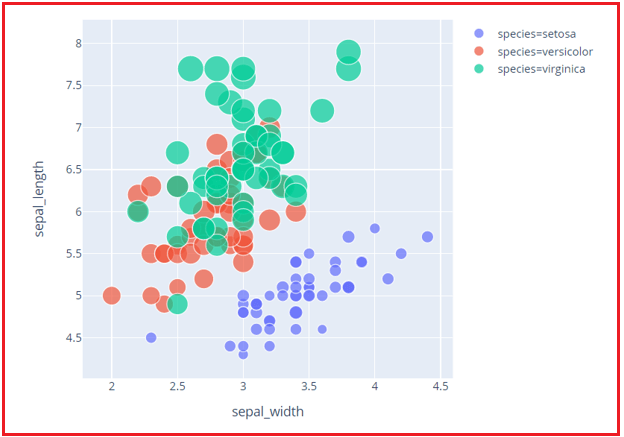# Scatter and Bubble Plots in Python using Plotly

## Scatter and Bubble Plots in Python using Plotly

In this article, I am going to discuss Scatter and Bubble Plots in Python using Plotly for Data Science with Examples. Please read our previous article where we discussed Line Charts in Python using Plotly for Data Science with Examples.

##### Scatter and Bubble Plots in Python using Plotly

The scatter diagram plots two sets of numerical data, one on each axis, to see if there’s a link between them. Each data point is represented by a marker point in px.scatter, whose location is determined by the x and y columns.

###### Example –
```# x and y scatter plot
import plotly.express as px
fig = px.scatter(x=[0, 1, 2, 3, 4], y=[0, 1, 4, 9, 16])
fig.show()
```
###### Output:###### Example of Scatter Plot for a dataset –
```# Scatter Plot for Iris Data
import plotly.express as px
data = px.data.iris() # iris is a pandas DataFrame
figure = px.scatter(data, x="sepal_width", y="sepal_length")
figure.show()
```
###### Output:###### Example of Scatter Plot for a dataset point differentiated category-wise –
```# x and y given as DataFrame columns
import plotly.express as px

# iris is a pandas DataFrame
data = px.data.iris()
# scatter plot category-wise
figure = px.scatter(data, x="sepal_width", y="sepal_length", color="petal_length")
figure.show()
```
###### Output:##### Bubble Plots in Python using Plotly

The scatter diagram plots two sets of numerical data, one on each axis, to see if there’s a link between them. Each data point is represented by a marker point in px.scatter, whose location is determined by the x and y columns.

###### Example –
```# x and y given as DataFrame columns
import plotly.express as px

# iris is a pandas DataFrame
data = px.data.iris()
# bubble chart for checking correlation between
# sepal_length and sepal_width according to petal length for different species
figure = px.scatter(data, x="sepal_width", y="sepal_length", color="species",
size='petal_length')
figure.show()
```
###### Output:In the next article, I am going to discuss Bar Charts in Python using Plotly for Data Science with Examples. Here, in this article, I try to explain Scatter and Bubble Plots in Python using Plotly for Data Science with Examples. I hope you enjoy this Scatter and Bubble Plots in Python using Plotly for Data Science article.